# Handles QuickSort and all of its methods. : Sort Search « Collections Data Structure « Java

Handles QuickSort and all of its methods.

```
//package sortcomparisons;

import java.util.Random;

/**
* Handles QuickSort and all of its methods.
*
* @author Alex Laird
* @version 1.0
*/
public class QuickSort
{
// object pointer delcarations
private static final Random random = new Random();

/**
* Swaps the two indeces in the array given.
*
* @param array The array to perform the swap on.
* @param first The first index to swap.
* @param second The second index to swap.
*/
private void swap(int[] array, int first, int second)
{
// swap array[i] and array[j]
int temp = array[first];
array[first] = array[second];
array[second] = temp;
}

/**
* Finds the most logical point to split the array in two parts and uses
* that point as the partition. Uses a for loop to evaluate the partition.
*
* @param array The array to be sorted.
* @param low The lowest index.
* @param high The highest index.
* @return The index of the partitioning point.
*/
private int partitionUsingFor(int[] array, int low, int high)
{
// get the value of the pivot element
int pivot = array[high];
// the low pointer
int i = low - 1;

for(int j = low; j < high; ++j)
{
// if the value at the current index in the array is smaller than the pivot, swap them
if(array[j] <= pivot)
{
i = ++i;

// swap array[i] and array[j]
swap(array, i, j);
}
}

// increment the low pointer
++i;

// swap array[i] and array[high]
swap(array, i, high);

return i;
}

/**
* Finds the most logical point to split the array in two parts and uses
* that point as the partition. Uses a while loop to evaluate the partition.
*
* @param array The array to be sorted.
* @param low The lowest index.
* @param high The highest index.
* @return The index of the partitioning point.
*/
private int partitionUsingWhile(int[] array, int low, int high)
{
int pivot = array[low];
int left = low;
int right = high;

while(left < right)
{
// increment the low pointer until you meet the pivot
while(left <= right && array[left] <= pivot)
{
++left;
}
// decrement the high pointer until you meet the pivot
while(left <= right && array[right] > pivot)
{
--right;
}

// if the pointers have crossed, swap the items
if(left < right)
{
// swap array[left] with array[right]
swap(array, left, right);
}
}

array[low] = array[right];
array[right] = pivot;

return right;
}

/**
* Generates the random partition location and calls the partition method.
* Uses a for loop to evaluate the partition.
*
* @param array The array to be sorted.
* @param low The lowest index.
* @param high The highest index.
* @return The index of the randomly generated partition.
*/
private int randomizedPartitionUsingFor(int[] array, int low, int high)
{
// some random int between low and high
int i = random.nextInt(high - low + 1) + low;

// swap array[high] and array[i]
swap(array, high, i);

return partitionUsingFor(array, low, high);
}

/**
* Generates the random partition location and calls the partition method.
* Uses a while loop to evaluate the partition.
*
* @param array The array to be sorted.
* @param low The lowest index.
* @param high The highest index.
* @return The index of the randomly generated partition.
*/
private int randomizedPartitionUsingWhile(int[] array, int low, int high)
{
// some random int between low and high
int i = random.nextInt(high - low + 1) + low;

// swap array[high] and array[i]
swap(array, high, i);

return partitionUsingWhile(array, low, high);
}

/**
* Performs a recursive QuickSort algorithm on an array from low to high, but
* does so by selecting randomized indeces for the partitions. Uses a for
* loop to evaluate the partition.
*
* @param array The array to be sorted.
* @param low The lowest index.
* @param high The highest index.
*/
public void sortRandomizedPartitionUsingFor(int[] array, int low, int high)
{
if(low < high)
{
// randomly locate a partition point
int mid = randomizedPartitionUsingFor(array, low, high);
// recursively sort the lower portion
sortRandomizedPartitionUsingFor(array, low, mid - 1);
// recursively sort the upper portion
sortRandomizedPartitionUsingFor(array, mid + 1, high);
}
}

/**
* Performs a recursive QuickSort algorithm on an array from low to high, but
* does so by selecting randomized indeces for the partitions. Uses a while
* loop to evaluate the partition.
*
* @param array The array to be sorted.
* @param low The lowest index.
* @param high The highest index.
*/
public void sortRandomizedPartitionUsingWhile(int[] array, int low, int high)
{
if(low < high)
{
// randomly locate a partition point
int mid = randomizedPartitionUsingWhile(array, low, high);
// recursively sort the lower portion
sortRandomizedPartitionUsingWhile(array, low, mid - 1);
// recursively sort the upper portion
sortRandomizedPartitionUsingWhile(array, mid + 1, high);
}
}

/**
* Performs a standard recursive QuickSort algorithm on an array from high
* to low. Uses a for loop to evaluate the partition.
*
* @param array The array to be sorted.
* @param low The lowest index.
* @param high The highest index.
*/
public void sortUsingFor(int[] array, int low, int high)
{
if(low < high)
{
// locate the most precise partition point
int mid = partitionUsingFor(array, low, high);
// recursively sort the lower half
sortUsingFor(array, low, mid - 1);
// recursively sort the upper half
sortUsingFor(array, mid + 1, high);
}
}

/**
* Performs a standard recursive QuickSort algorithm on an array from high
* to low. Uses a while loop to evaluate the partition.
*
* @param array The array to be sorted.
* @param low The lowest index.
* @param high The highest index.
*/
public void sortUsingWhile(int[] array, int low, int high)
{
if(low < high)
{
// locate the most precise partition point
int mid = partitionUsingWhile(array, low, high);
// recursively sort the lower half
sortUsingWhile(array, low, mid - 1);
// recursively sort the upper half
sortUsingWhile(array, mid + 1, high);
}
}
}

```

### Related examples in the same category

 1 Linear search 2 Animation for quick sort 3 Quick Sort Implementation with median-of-three partitioning and cutoff for small arrays 4 Simple Sort Demo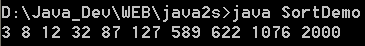5 A simple applet class to demonstrate a sort algorithm 6 Sorting an array of Strings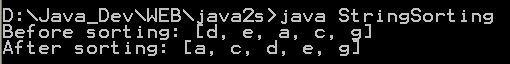7 Simple version of quick sort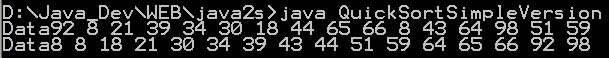8 Combine Quick Sort Insertion Sort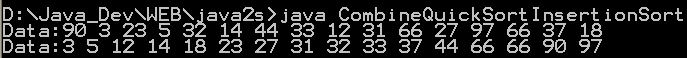9 Quick sort with median-of-three partitioning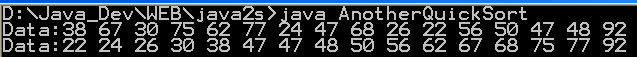10 Fast Quick Sort 11 Selection sort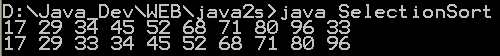12 Insert Sort for objects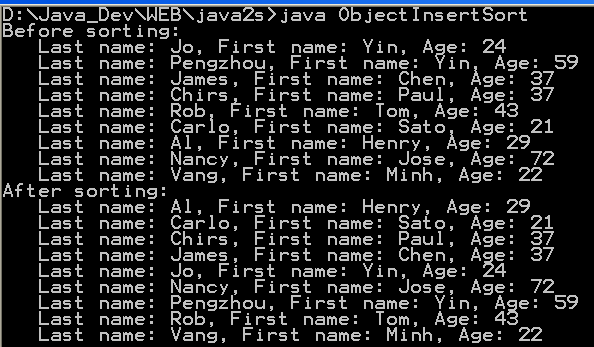13 Insert sort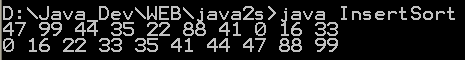14 Bubble sort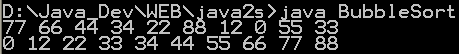15 Merge sort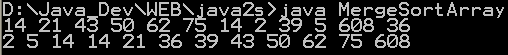16 Fast Merge Sort 17 Binary Search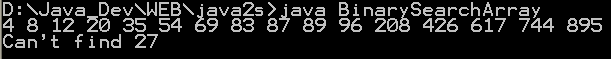18 Shell sort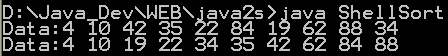19 Recursive Binary Search Implementation in Java 20 Topological sorting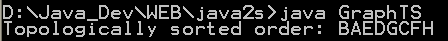21 Heap sort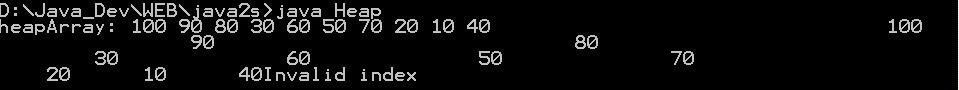22 Sort Numbers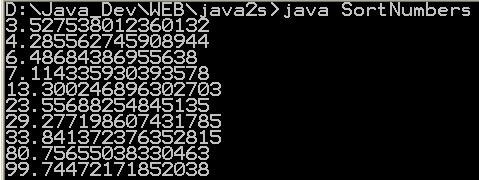23 A quick sort demonstration algorithm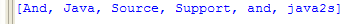24 Performing Binary Search on Java byte Array Example 25 Performing Binary Search on Java char Array Example 26 Performing Binary Search on Java double Array Example 27 Performing Binary Search on Java float Array Example 28 Performing Binary Search on Java int Array Example 29 Performing Binary Search on Java long Array Example 30 Performing Binary Search on Java short Array 31 Sort items of an array 32 Sort an array of objects 33 Sort a String array 34 Sort string array with Collator 35 Binary search routines 36 FastQ Sorts the [l,r] partition (inclusive) of the specfied array of Rows, using the comparator.37 A binary search implementation. 38 Implements QuickSort three different ways 39 A quick sort algorithm to sort Vectors or arrays. Provides sort and binary search capabilities.40 Returns an array of indices indicating the order the data should be sorted in.